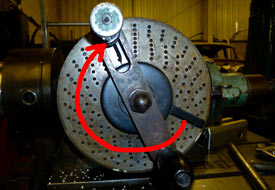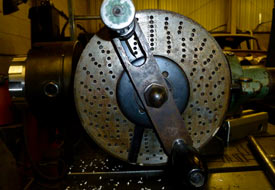####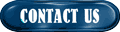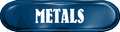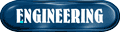####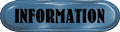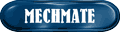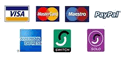###How to use a divide head and rotary table.

The divide head and rotary table are a device used to rotate the work piece around a third machine axis, this is usually referred to as the 'C' axis and is commonly used on milling machines or borers to provide the ability to easily create accurate single or multiple machining operations around a component.

A typical use would be to quickly machine a hexagon profile around a diameter, or to produce a series of cut-outs at a specific pitch from one another. The divide head is similar to that of mounting a lathe head on a milling machine table to produce a horizontal rotating axis, where as a rotary table is more commonly used to rotate the work piece vertically. Of course both devices may be mounted then moved in multiple orientations besides that mentioned above in order to produce the profile required.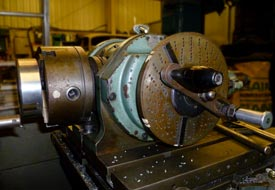Movement of the device is a simple matter of rotating a handle in the desired direction until the degree of movement is obtained that you need to rotate the part. In simple use, the engineer would mark-off the work piece prior to mounting onto the 'C' axis, then having mounted a centre drill or pointer into the machine chuck - rotate the part until the markings align central to the spindle.

Of course this method is only as accurate as ones marking-off and sight allows! Some cheaper devices are fitted with only a graduated dial for rotational movement, but for perfect precision the machinist must make use of the 'Hole Plate', which is an integral part of most 'older' or better quality dividing heads and rotary tables.

### HOW TO USE THE HOLE PLATE

The hole plate is a static circular plate mounted to the rear of the rotation handle which is pre-drilled with many equally spaced holes at a different series of pitches. The pitch decreases as one moves from the centre of the hole plate to the outer edge (made clearer on the photo to the right). The axis handle has both a sprung pin - which may be inserted into one of the holes as well as a set of ‘clock hands’ and pointers that aid repetitive movements. (we will come back to the pointers later in this paper)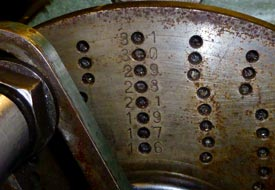The ratio of turns between the device handle and the chuck (or table) is always 40:1, so 40 turns of the device handle would give 1 complete turn of the chuck. This means that one rotation of the handle would produce 9 degrees of chuck movement.

Now, each 9 degrees is then split into a number of graduations - the holes on the hole plate. Marked on your device hole plate will be number that corresponds to the numbers of holes are drilled on a certain area of the hole plate. These numbers are to be used to quickly identify if the degree of chuck movement to produce the desired division can be achieved on one set of drilled holes. (I know this sounds complicated but hopefully the example below will clear the method up a bit - if you have problems with the calculation then try our on-line hole plate calculator).

### EXAMPLE AND METHOD 1

In this example we will produce a set of 7 slots, equally spaced all around a diameter.

The first thing to do is to work out the number of decimal degrees for the spacing of the slots, so that is 360 degrees divided by 7 divisions.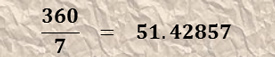Next divide the result between 40 turns of the handle to give the number of turns per division.

That equals 5.71428 turns of the handle for each of the 7 divisions, which is roughly five and three quarter turns.Next we must work out the total number of holes on each series that are on the hole plate. So, for a series of 19 holes - this would be: 19 holes X 40 turns = 760 holes per 360 degrees of rotation of the chuck. (remember that all dividing heads are at 40:1 ratio so you need to turn the handle 40 times to produce 360 degrees of movement at the chuck).

Listed to the right is all of the common hole plate series with the total number of holes per series already calculated for ease of following this tutorial.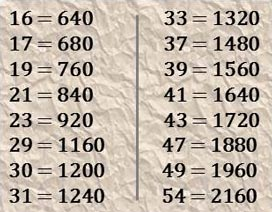We must now find out which series of holes will exactly match the angular movement that we need. This is done by dividing the total number of holes on a given hole series by 360 degrees. This result must then be further divided by the result of our first calculation, which in this case is 51.42857.

The purpose of this final calculation is to obtain a resulting whole number by applying different total holes per series into the calculation. ie, for 37 hole series - 1480 must be inserted into the equation, or for 21 hole series, 840 should be used. If a whole number can not be achieved then a hole series must be used with the least amount of error after the decimal point. ie, 120.009 would be a better selection than 120.01)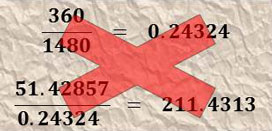This process may seem at first to be somewhat 'trial and error', but after repeating the process several times the engineer will gain enough experience to be able to make an educated guess at the likely hole series to use.

One should also bear in mind that it is entirely possible that several hole plate series may be used to produce a given angular movement.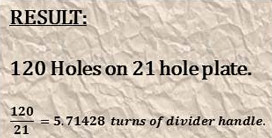### EXAMPLE AND METHOD 2

We will now calculate the hole series to use to produce 21 divisions on a diameter. In this example we will use this formulae to aid with the math.

It is always worth the time to work out the number of handle turns (t) as this will provide a guide of the handle movement to expect from the calculation, this number should be used also at the end of the equation to verify the result (rule).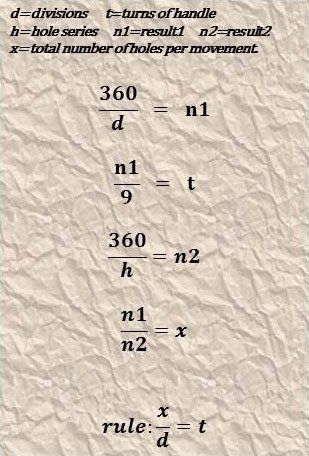(n1) We need 21 divisions, so 360 degrees divided by 21 divisions.(t) Calculate the number of handle turns to check final result.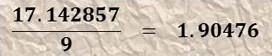(n2) Insert total number of holes of different series of hole plates.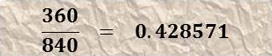(x) Divide first result over the answer until whole number is achieved by substituting different hole plate series results into calculation.(rule) Record the result for use with dividing head and check math by dividing the resulting number of holes over the hole plate series. The figure should equal the number of handle turns calculated above.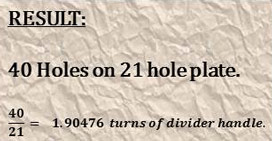Remember that it is entirely possible to use any of several hole plate series to achieve the same result and that the hole series resulting in the closest whole number should always be preferable for use for the most accurate and precise angular movement.

Our on-line calculator can help the hole plate selection process, all you need is to know the series of holes that you have available on your hole plate. Give it a try by clicking on the 'calculators' link.

### USING THE 'CLOCK HANDS'

Most dividers are fitted with a set of 'clock hands', or pointers that are designed to aid quick location of the handle pin into a hole in the hole plate. These can be set to a specific number of holes about the hole plate to ensure that the engineer does not loose count of the holes during a chuck movement.

The pointers are adjustable to rotate around the centre and can be moved independantly as desired via releasing the locking screw. This can be found by gently rotating the centre cover until a screw head can be seen through a small inspection hole drilled into the cover.Utilising the clock hands is achieved by aligning the first hand against the front of the handle pin, then counting the number of holes required to obtain the correct angular movement and setting the second hand about this hole location.

For example: using a 21 series hole plate, we know that we need 40 holes. So, set the first pointer against the front of the handle pin - then move the second pointer 2 holes anti-clockwise about the plate and secure the locking screw. Perform the machining operation at this position then move the clock hands as a complete unit (both hands are now locked together via the locking screw) so that the second hand now touches the rear of the handle pin. Move the handle clockwise so that the handle pin is against the rear of the first pointer (one full rotation and nineteen holes - 21 holes + 19 holes = 40 holes). Continue this procedure until the machining operations are complete about the full 360 degree movement.

It is important to remember to continue the rotational movements in the same direction about the entire machining operation. This way any backlash in the mechanism of the rotaty axis will not effect the finished product.